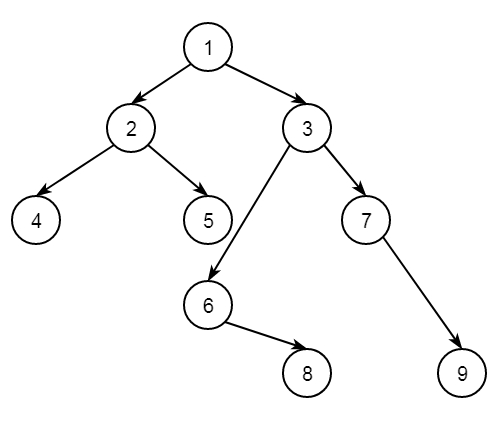# Find the kth node in vertical order traversal of a Binary Tree in C++

C++Server Side ProgrammingProgramming

Suppose we have a binary tree and a value K. The task is to print the Kth node in the vertical order traversal. If no such node exists, then return -1. So if the tree is like below −The vertical order traversal is like −

4
2
1 5 6
3 8
7
9

So if K = 3, then result will be 1.

The approach is simple. We will perform the vertical order traversal, then check the current node is the kth node or not, if so then return.

## Example

Live Demo

#include<iostream>
#include<map>
#include<vector>
#include<queue>
using namespace std;
class Node {
public:
int key;
Node *left, *right;
};
Node* getNode(int key){
Node* node = new Node;
node->key = key;
node->left = node->right = NULL;
return node;
}
int findKthNodeVertical(Node* root, int k) {
if (!root || k == 0)
return -1;
int n = 0;
int k_node = -1;
map<int, vector<int> > current_map;
int hd = 0;
queue<pair<Node*, int> > que;
que.push(make_pair(root, hd));
while (!que.empty()) {
pair<Node*, int> temp = que.front();
que.pop();
hd = temp.second;
Node* node = temp.first;
current_map[hd].push_back(node->key);
if (node->left != NULL)
que.push(make_pair(node->left, hd - 1));
if (node->right != NULL)
que.push(make_pair(node->right, hd + 1));
}
map<int, vector<int> >::iterator it;
for (it = current_map.begin(); it != current_map.end(); it++) {
for (int i = 0; i < it->second.size(); ++i) {
n++;
if (n == k)
return (it->second[i]);
}
}
if (k_node == -1)
return -1;
}
int main() {
Node* root = getNode(1);
root->left = getNode(2);
root->right = getNode(3);
root->left->left = getNode(4);
root->left->right = getNode(5);
root->right->left = getNode(6);
root->right->right = getNode(7);
root->right->left->right = getNode(8);
root->right->right->right = getNode(9);
int k = 3;
cout << "Kth node in vertical traversal: " << findKthNodeVertical(root, k);
}

## Output

Kth node in vertical traversal: 1